Main Content

# hornConical

Create conical horn antenna

## Description

The `hornConical` object creates a waveguide shaped like a cone to direct radio waves in a beam. This type of horn is widely used as feed element for large radio astronomy telescopes, satellite tracking, and communication dishes.## Creation

### Syntax

``ant = hornConical``
``ant = hornConical(Name,Value)``

### Description

example

````ant = hornConical` creates a conical horn antenna with dimensions for an operating frequency of 7.58 GHz.```

example

````ant = hornConical(Name,Value)` sets properties using one or more name-value pairs. For example, `ant = hornConical('Radius',1)` creates a conical horn antenna with a radius of 1 meter.```

## Properties

expand all

Radius of the waveguide, specified as a real-valued scalar in meters.

Example: `'Radius',0.760`

Example: `ant.Radius = 0.760`

Data Types: `double`

Height of the waveguide, specified as a real-valued scalar in meters.

Example: `'WaveguideHeight',0.0340`

Example: `ant.WaveguideHeight = 0.0340`

Data Types: `double`

Height of the feed, specified as a real-valued scalar in meters.

Example: `'FeedHeight',0.0085`

Example: `ant.FeedHeight = 0.0085`

Data Types: `double`

Width of the feed, specified as a real-valued scalar in meters.

Example: `'FeedWidth',0.0200`

Example: `ant.FeedWidth = 0.0200`

Data Types: `double`

Signed distances along the Y-axis, specified as a real-valued scalar in meters.

Example: `'FeedOffset',0.03627`

Example: `ant.FeedOffset = 0.3627`

Data Types: `double`

Height of the cone, specified as a real-valued scalar in meters.

Example: `'ConeHeight',0.0540`

Example: `ant.ConeHeight = 0.0540`

Data Types: `double`

Radius of the cone aperture, specified as a real-valued scalar in meters.

Example: `'ApertureRadius',0.0760`

Example: `ant.ApertureRadius = 0.0760`

Data Types: `double`

Type of the metal used as a conductor, specified as a metal material object. You can choose any metal from the `MetalCatalog` or specify a metal of your choice. For more information, see `metal`. For more information on metal conductor meshing, see Meshing.

Example: ```m = metal('Copper'); 'Conductor',m```

Example: ```m = metal('Copper'); ant.Conductor = m```

Lumped elements added to the antenna feed, specified as a lumped element object handle. You can add a load anywhere on the surface of the antenna. By default, the load is at the feed. For more information, see `lumpedElement`.

Example: `'Load',lumpedelement`. `lumpedelement` is the object handle for the load created using `lumpedElement`.

Example: ```ant.Load = lumpedElement('Impedance',75)```

Tilt angle of the antenna, specified as a scalar or vector with each element unit in degrees. For more information, see Rotate Antennas and Arrays.

Example: `'Tilt',90`

Example: `ant.Tilt = 90`

Example: `'Tilt',[90 90]`,`'TiltAxis',[0 1 0;0 1 1]` tilts the antenna at 90 degrees about the two axes defined by the vectors.

Note

The `wireStack` antenna object only accepts the dot method to change its properties.

Data Types: `double`

Tilt axis of the antenna, specified as:

• Three-element vector of Cartesian coordinates in meters. In this case, each coordinate in the vector starts at the origin and lies along the specified points on the X-, Y-, and Z-axes.

• Two points in space, each specified as three-element vectors of Cartesian coordinates. In this case, the antenna rotates around the line joining the two points in space.

• A string input describing simple rotations around one of the principal axes, 'X', 'Y', or 'Z'.

For more information, see Rotate Antennas and Arrays.

Example: `'TiltAxis',[0 1 0]`

Example: `'TiltAxis',[0 0 0;0 1 0]`

Example: `ant.TiltAxis = 'Z'`

Note

The `wireStack` antenna object only accepts the dot method to change its properties.

Data Types: `double`

## Object Functions

 `show` Display antenna or array structure; display shape as filled patch `axialRatio` Axial ratio of antenna `beamwidth` Beamwidth of antenna `charge` Charge distribution on metal or dielectric antenna or array surface `current` Current distribution on metal or dielectric antenna or array surface `design` Design prototype antenna or arrays for resonance at specified frequency `efficiency` Radiation efficiency of antenna `EHfields` Electric and magnetic fields of antennas; Embedded electric and magnetic fields of antenna element in arrays `impedance` Input impedance of antenna; scan impedance of array `mesh` Mesh properties of metal or dielectric antenna or array structure `meshconfig` Change mesh mode of antenna structure `optimize` Optimize antenna or array using SADEA optimizer `pattern` Radiation pattern and phase of antenna or array; Embedded pattern of antenna element in array `patternAzimuth` Azimuth pattern of antenna or array `patternElevation` Elevation pattern of antenna or array `returnLoss` Return loss of antenna; scan return loss of array `sparameters` S-parameter object `vswr` Voltage standing wave ratio of antenna

## Examples

collapse all

Create and view a default conical horn antenna.

`ant = hornConical`
```ant = hornConical with properties: Radius: 0.0120 WaveguideHeight: 0.0300 FeedHeight: 0.0075 FeedWidth: 0.0030 FeedOffset: 0.0100 ConeHeight: 0.0348 ApertureRadius: 0.0350 Conductor: [1x1 metal] Tilt: 0 TiltAxis: [1 0 0] Load: [1x1 lumpedElement] ```
`show(ant)`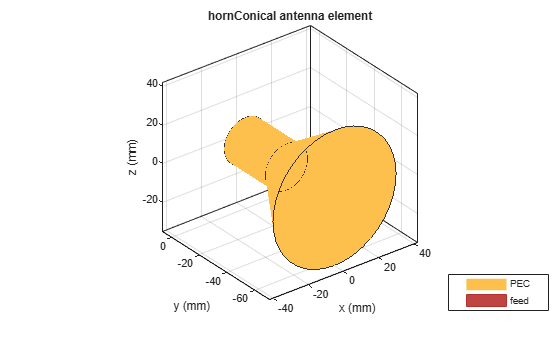Plot the radiation pattern of the antenna at 7.58 GHz.

`pattern(ant,7.58e9)`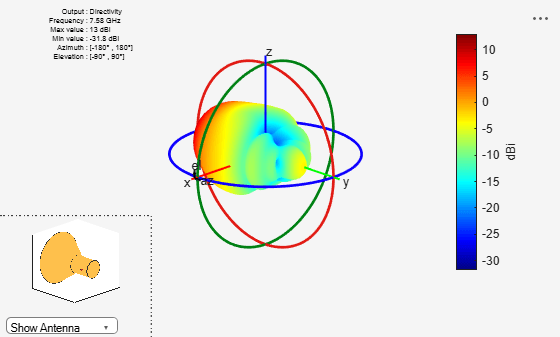Create a conical horn antenna with the following dimensions.

```ant=hornConical('Radius',35.71e-3,'WaveguideHeight',200e-3,... 'Feedwidth',26e-3,'FeedHeight',34.71e-3,'FeedOffset',42.42e-3,... 'ConeHeight',130e-3,'ApertureRadius',62.5e-3); show(ant);```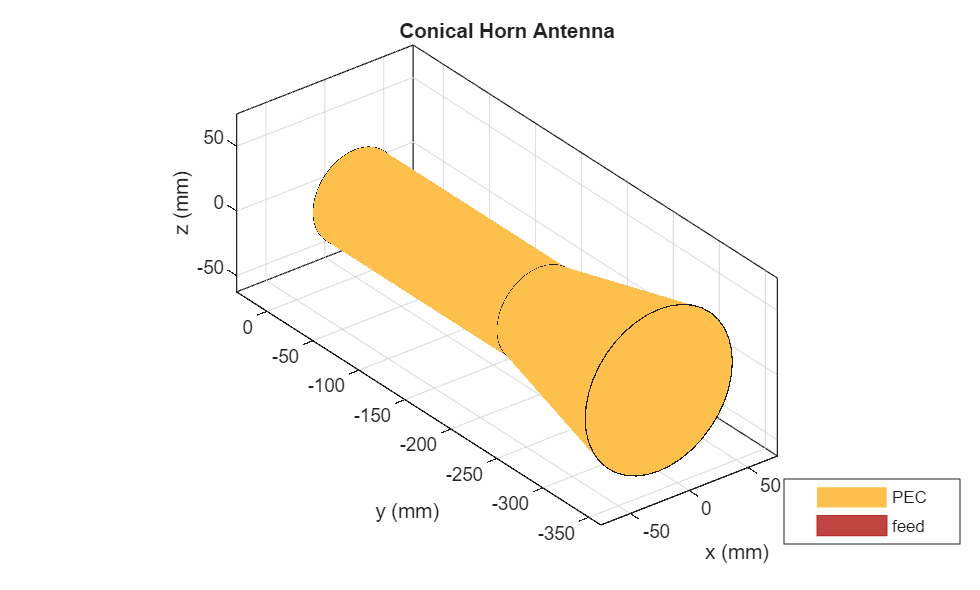Plot the s-parameters and the impedance of the antenna.

```s=sparameters(ant,2.5e9:20e6:4e9); rfplot(s);```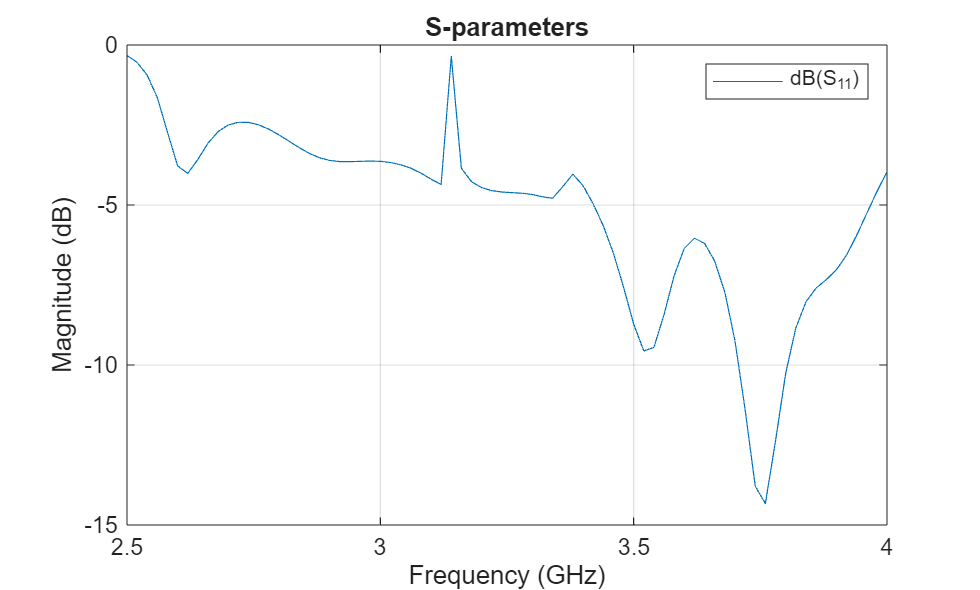```figure; impedance(ant,2.5e9,20e6:4e9); ```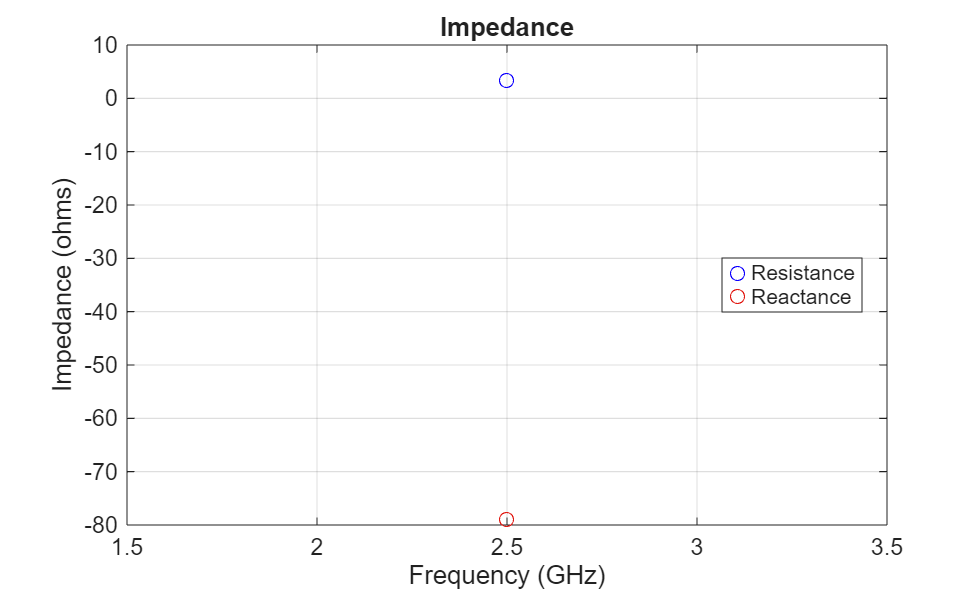Jadhav, Rohini.P, Vinithkurnar Javnrakash Dongre, Arunkumar Heddallikar. "Design of X-Band Conical Horn Antenna Using Coaxial Feed and Improved Design Technique for Bandwidth Enhancement." In International Conference on Computing, Communication, Control, and Automation (ICCUBEA), 1-6. Pune, India: ICCUBEA 2017

## Support

#### Hybrid Beamforming for Massive MIMO Phased Array Systems

Download the white paper